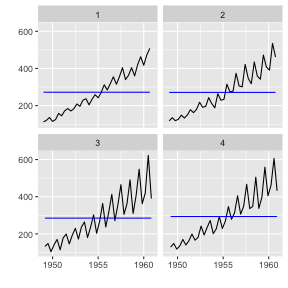This document explains time series related plotting using ggplot2 and {ggfortify}.

Plotting ts objects

{ggfortify} let {ggplot2} know how to interpret ts objects. After loading {ggfortify}, you can use ggplot2::autoplot function for ts objects.

library(ggfortify)
autoplot(AirPassengers)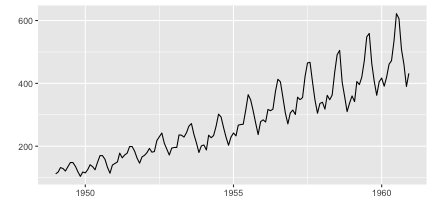To change line colour and line type, use ts.colour and ts.linetype options. Use help(autoplot.ts) (or help(autoplot.*) for any other objects) to check available options.

autoplot(AirPassengers, ts.colour = 'red', ts.linetype = 'dashed')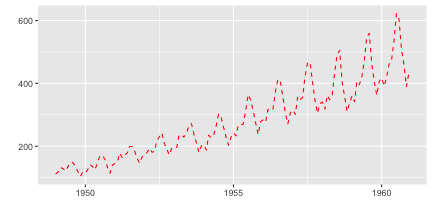Multivariate time series will be drawn with facets.

library(vars)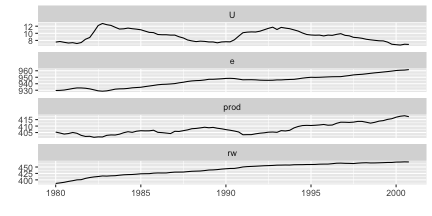Specify facets = FALSE to draw on single axes.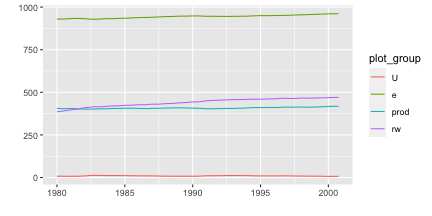Also, autoplot can handle other time-series-likes. Supported packages are:

• zoo::zooreg
• xts::xts
• timeSeries::timSeries
• tseries::irts
library(xts)
autoplot(as.xts(AirPassengers), ts.colour = 'green')

library(timeSeries)
autoplot(as.timeSeries(AirPassengers), ts.colour = ('dodgerblue3'))

Specifying geometrics

You can change {ggplot2} geometrics specifying by its name. Geometrics currently supported are line, bar, ribbon and point.

autoplot(AirPassengers, ts.geom = 'bar', fill = 'blue')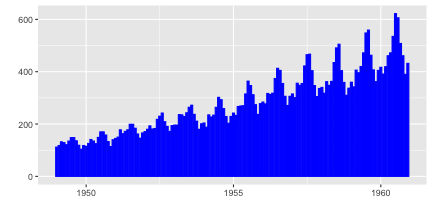autoplot(AirPassengers, ts.geom = 'ribbon', fill = 'green')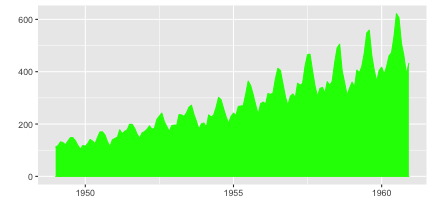autoplot(AirPassengers, ts.geom = 'point', shape = 3)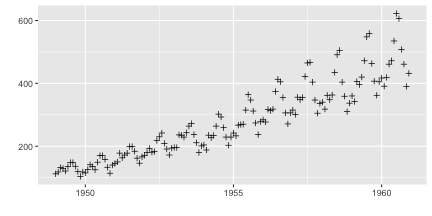As described above, multivariate timeseries can be drawn in a single grid specifying facets = FALSE. Time series are not stacked by default. Specifying stacked = TRUE allows stacking.

mts <- ts(data.frame(a = c(1, 2, 3, 4, 4, 3), b = c(3, 2, 3, 2, 2, 1)), start = 2010)
autoplot(mts, ts.geom = 'bar', facets = FALSE)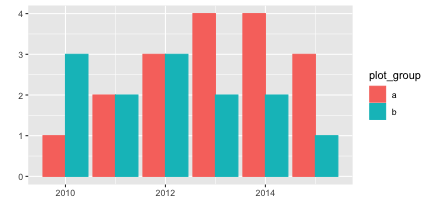autoplot(mts, ts.geom = 'bar', facets = FALSE, stacked = TRUE)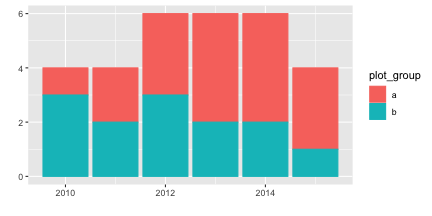autoplot(mts, ts.geom = 'ribbon', facets = FALSE)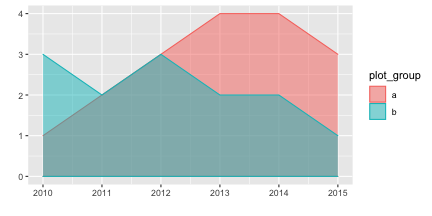autoplot(mts, ts.geom = 'ribbon', facets = FALSE, stacked = TRUE)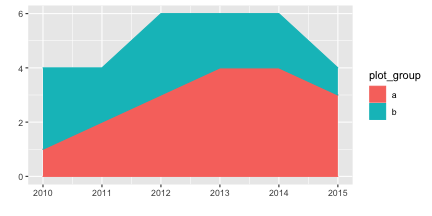Plotting with forecast package

{ggfortify} supports forecast object in the {forecast} package.

library(forecast)
d.arima <- auto.arima(AirPassengers)
d.forecast <- forecast(d.arima, level = c(95), h = 50)
autoplot(d.forecast)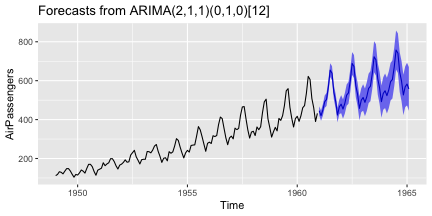There are some options to change basic settings.

autoplot(d.forecast, ts.colour = 'firebrick1', predict.colour = 'red',
predict.linetype = 'dashed', conf.int = FALSE)Plotting with vars package

ggfortify supports varpred object in vars package.

library(vars)
d.vselect <- VARselect(Canada, lag.max = 5, type = 'const')\$selection
d.var <- VAR(Canada, p = d.vselect, type = 'const')

Available options are the same as forecast.

autoplot(predict(d.var, n.ahead = 50), ts.colour = 'dodgerblue4',
predict.colour = 'blue', predict.linetype = 'dashed')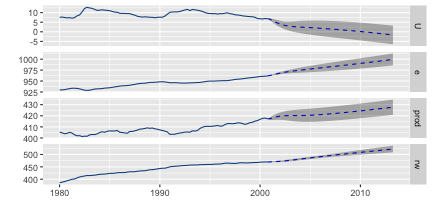Plotting with changepoint package

{ggfortify} supports cpt object in {changepoint} package.

library(changepoint)
autoplot(cpt.meanvar(AirPassengers))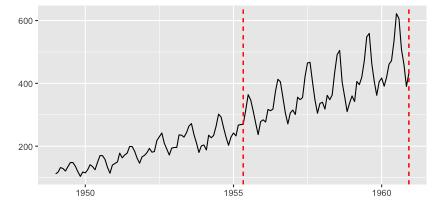You can change some options for cpt.

autoplot(cpt.meanvar(AirPassengers), cpt.colour = 'blue', cpt.linetype = 'solid')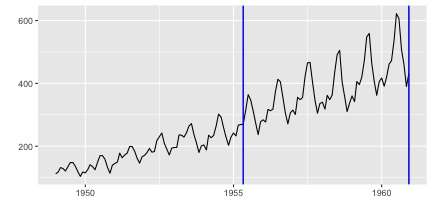Plotting with strucchange package

ggfortify supports breakpoints object in strucchange package. Same plotting options as changepoint are available.

library(strucchange)
autoplot(breakpoints(Nile ~ 1), ts.colour = 'blue', ts.linetype = 'dashed',
cpt.colour = 'dodgerblue3', cpt.linetype = 'solid')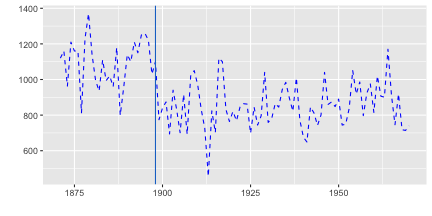Plotting with {dlm} package

autoplot can draw both original and fitted time series, because dlm::dlmFilter contains them.

library(dlm)
form <- function(theta){
dlmModPoly(order = 1, dV = exp(theta), dW = exp(theta))
}

model <- form(dlmMLE(Nile, parm = c(1, 1), form)\$par)
filtered <- dlmFilter(Nile, model)

autoplot(filtered)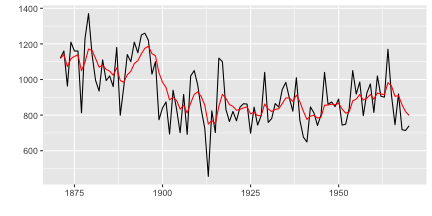You can specify some options to change how plot looks, such as ts.linetype and fitted.colour. Use help(autoplot.tsmodel) (or help(autoplot.*) for any other objects) to check available options.

autoplot(filtered, ts.linetype = 'dashed', fitted.colour = 'blue')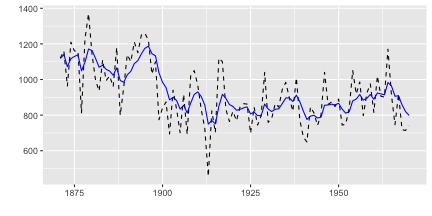{ggfortify} can plot dlm::dlmSmooth instance which returns a list instance using using class inference. Note that only smoothed result is plotted because dlm::dlmSmooth doesn't contain original ts.

smoothed <- dlmSmooth(filtered)
class(smoothed)
##  "list"
autoplot(smoothed)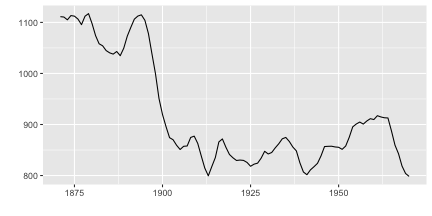To plot original ts, filtered result and smoothed result, you can useautoplot as below. When autoplot accepts ggplot instance via p option, continuous plot is drawn on the passed ggplot.

p <- autoplot(filtered)
autoplot(smoothed, ts.colour = 'blue', p = p)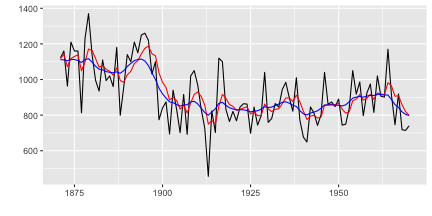Plotting with {KFAS} package

You can use autoplot in almost the same manner as {dlm}. Note that autoplot draws smoothed result if it exists in KFAS::KFS instance, and KFAS::KFS contains smoothed result by default.

library(KFAS)
model <- SSModel(
Nile ~ SSMtrend(degree=1, Q=matrix(NA)), H=matrix(NA)
)

fit <- fitSSM(model=model, inits=c(log(var(Nile)),log(var(Nile))), method="BFGS")
smoothed <- KFS(fit\$model)
autoplot(smoothed)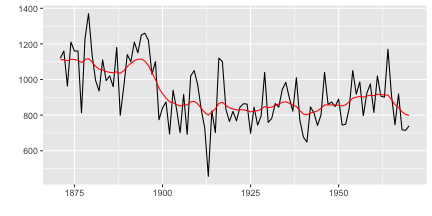If you want filtered result, specify smoothing='none' when calling KFS. For details, see help(KFS).

filtered <- KFS(fit\$model, filtering="mean", smoothing='none')
autoplot(filtered)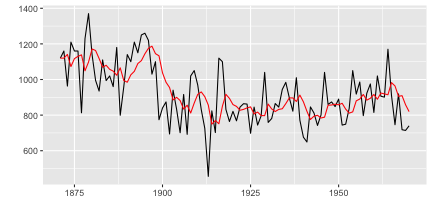Also, KFAS::signal will retrieve specific state from KFAS::KFS instance. The result will be a list which contains the retrieved state as ts in signal attribute. ggfortify can autoplot it using class inference.

trend <- signal(smoothed, states="trend")
class(trend)
##  "list"

Because signal is a ts instance, you can use autoplot and p option as the same as dlm::dlmSmooth example.

p <- autoplot(filtered)
autoplot(trend, ts.colour = 'blue', p = p)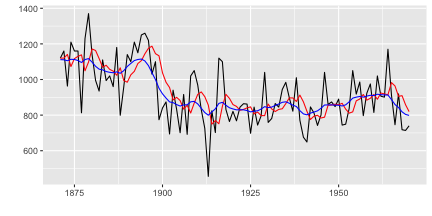Plotting time series statistics

{ggfortify} supports following time series related statistics in stats package:

• stl, decomposed.ts
• acf, pacf, ccf
• spec.ar, spec.pgram
• cpgram (covered by ggcpgram)
autoplot(stl(AirPassengers, s.window = 'periodic'), ts.colour = 'blue')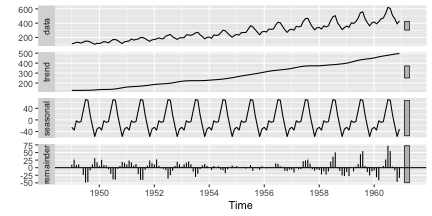NOTE With acf and spec.*, specify plot = FALSE to suppress default plotting outputs.

autoplot(acf(AirPassengers, plot = FALSE))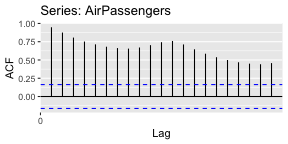You can pass some options when plotting acf via autoplot. Built-in acf calcurates the confidence interval at plotting time and doesn't hold the result, equivalent options can be passed to autoplot. Following example shows to change the value of confidence interval and method (use ma assuming the input follows MA model).

autoplot(acf(AirPassengers, plot = FALSE), conf.int.fill = '#0000FF', conf.int.value = 0.8, conf.int.type = 'ma')autoplot(spec.ar(AirPassengers, plot = FALSE))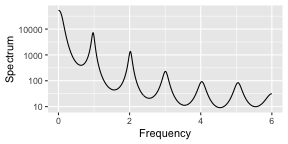ggcpgram should output the cumulative periodogram as the same as cpgram. Because cpgram doesn't have return value, we cannot use autoplot(cpgram(...)).

ggcpgram(arima.sim(list(ar = c(0.7, -0.5)), n = 50))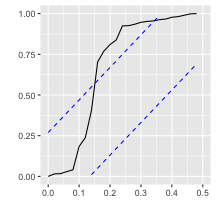ggtsdiag should output the similar diagram as tsdiag.

library(forecast)
ggtsdiag(auto.arima(AirPassengers))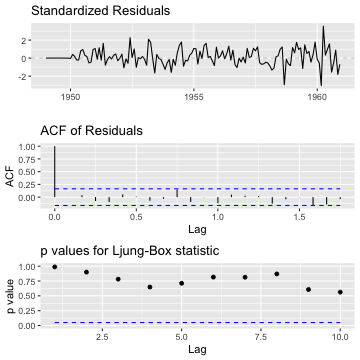ggfreqplot is a genelarized month.plot. You can pass freq if you want, otherwise time-series's frequency will be used.

ggfreqplot(AirPassengers)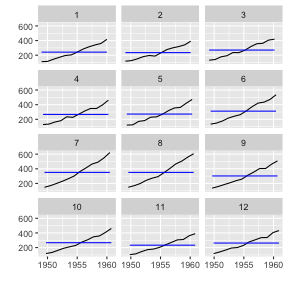ggfreqplot(AirPassengers, freq = 4)